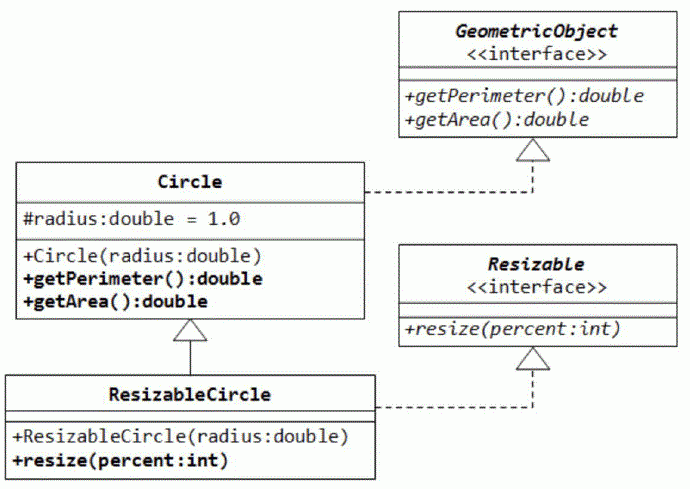Competitions

Java Geometric Object

Write an application for the following UML diagram:• Write the interface called GeometricObject, which declares two methods: getParameter() and getArea();
• Write the implementation class Circle, with a protected variable radius, which implements the interface GeometricObject;
• The class ResizableCircle is defined as a subclass of the class Circle, which also implements an interface called Resizable. The interface Resizable declares a method resize(), which modifies the dimension (such as radius) by the given percentage. Write the interface Resizable and the class ResizableCircle;
• Write a test program to test the methods defined in ResizableCircle.

Process the next instructions:

• Area - print the area of the circle;
• Perimeter - print the circumference of the circle;
• Resize percent - resize the radius of the circle that modifies the radius by the given percentage;

Initially the radius of the circle equals to 1.

Resize by 50 percent means decrease the radius twice. Resize by 200 percent means increase the radius twice.

Input

Each line contains one of the instructions:

• Area
• Perimeter
• Resize percent

Output

Print the output for each instruction.

Time limit 1 second
Memory limit 128 MiB
Input example #1
Area
Perimeter
Resize 50
Area
Perimeter
Output example #1
3.141593
6.283185
0.785398
3.141593
Author Michael Medvediev